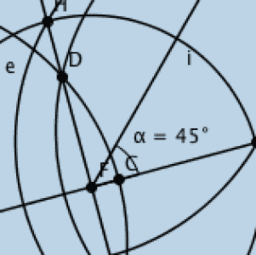# Constructions (Compass & Straightedge)

Strand 2: Geometry and Trigonometry Topic Description of topic Students learn about Learning outcomes Students should be able to 2.1 Synthetic geometry Concepts (see Geometry Course section 9.1 for OL and 10.1 for HL) Axioms (see Geometry Course section 9.3 for OL and 10.3 for HL): 1. [Two points axiom] There is exactly one line through any two given points. 2. [Ruler axiom] The properties of the distance between points 3. [Protractor Axiom] The properties of the degree measure of an angle 4. Congruent triangles (SAS, ASA and SSS) 5. [Axiom of Parallels] Given any line l and a point P, there is exactly one line through P that is parallel to l. Theorems: [Formal proofs are not examinable at OL. Formal proofs of theorems 4, 6, 9, 14 and 19 are examinable at HL.] 1. Vertically opposite angles are equal in measure. 2. In an isosceles triangle the angles opposite the equal sides are equal. Conversely, if two angles are equal, then the triangle is isosceles. 3. If a transversal makes equal alternate angles on two lines then the lines are parallel, (and converse). 4. The angles in any triangle add to 180˚. 5. Two lines are parallel if and only if, for any transversal, the corresponding angles are equal. 6. Each exterior angle of a triangle is equal to the sum of the interior opposite angles. 9. In a parallelogram, opposite sides are equal and opposite angles are equal (and converses). 10. The diagonals of a parallelogram bisect each other. 11. I f three parallel lines cut off equal segments on some transversal line, then they will cut off equal segments on any other transversal. 12. L et ABC be a triangle. If a line l is parallel to BC and cuts [AB] in the ratio s:t, then it also cuts [AC] in the same ratio (and converse). 13. If two triangles are similar, then their sides are proportional, in order (and converse). 14. [Theorem of Pythagoras] In a right-angled triangle the square of the hypotenuse is the sum of the squares of the other two sides. 15. If the square of one side of a triangle is the sum of the squares of the other two sides, then the angle opposite the first side is a right angle. 19. T he angle at the centre of a circle standing on a given arc is twice the angle at any point of the circle standing on the same arc. − recall the axioms and use them in the solution of problems − use the terms: theorem, proof, axiom, corollary, converse and implies − apply the results of all theorems, converses and corollaries to solve problems − prove the specified theorems Description of topic Students learn about Learning outcomes Students should be able to Corollaries: 1. A diagonal divides a parallelogram into 2 congruent triangles. 2. A ll angles at points of a circle, standing on the same arc, are equal, (and converse). 3. Each angle in a semi-circle is a right angle. 4. If the angle standing on a chord [BC] at some point of the circle is a right-angle, then [BC] is a diameter. 5. I f ABCD is a cyclic quadrilateral, then opposite angles sum to 180˚, (and converse). Constructions: 1. Bisector of a given angle, using only compass and straight edge. 2. Perpendicular bisector of a segment, using only compass and straight edge. 3. L ine perpendicular to a given line l, passing through a given point not on l. 4. Line perpendicular to a given line l, passing through a given point on l. 5. Line parallel to a given line, through a given point. 6. Division of a line segment into 2 or 3 equal segments, without measuring it. 7. Division of a line segment into any number of equal segments, without measuring it. 8. Line segment of a given length on a given ray. 9. Angle of a given number of degrees with a given ray as one arm. 10. Triangle, given lengths of three sides 11. Triangle, given SAS data 12. Triangle, given ASA data 13. Right-angled triangle, given the length of the hypotenuse and one other side. 14. Right-angled triangle, given one side and one of the acute angles (several cases). 15. Rectangle, given side lengths. − complete the constructions specified